UpValues

Normally Cosh[x]+Sinh[x] doesn't evaluate to Exp[x] but there are different
ways of making the kernel automatically perform this simplification.  A few
ways to do this are discussed in the cells below.  Before we begin the next
cell should be evaluated to unprotect the functions that will be modified.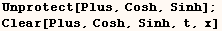Once Plus is unprotected evaluating the next cell will cause an expression  such as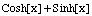to evaluate to Exp[z].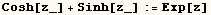The rule above has the desired effect in the cell below.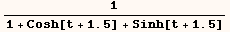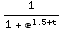However, Plus is a function that's used very often, and the code above  will slow down evaluation of Plus.  Actually it doesn't slow down Plus as  much as one might think.  In  Mathematica versions 3 and 4 the functions Plus and Times apply built in rules before  user defined rules.  So when the next cell evaluates the kernel uses a  built-in rule to compute (2+3) before it checks for the above user defined  identity.  Likewise built-in rules are used for (3+2/3), and (x+2 x), so here  too the kernel never gets a chance to see if the user defined rule above  should be used.  As a result the user defined rule had no effect on the speed  of evaluating the cell below.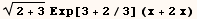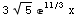On the other hand consider evaluation of the next cell after the above
identity for Cosh[z_]+Sinh[z_] is in the kernel.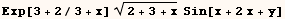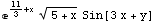When evaluating the cell above the kernel uses a built-in rule to evaluate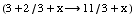, then it checks to see if the user defined rule for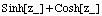applies to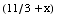.  Later in the evaluation the kernel uses a built-in rule to evaluate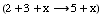and it checks to see if the user defined rule for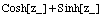applies to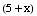.  Still later in the evaluation the kernel uses a built-in rule to  evaluate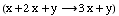and it checks to see if the user defined rule forapplies to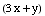.  In this case the user defined rule forcauses degradation in the time it takes to evaluate the last example even  though the functions Cosh, Sinh appear nowhere in the expression.

The user  defined rule above was a "DownValue" for Plus as can be seen from the next  cell.  When the rule was entered above the kernel determined that Plus is the  head ofand that is why it was associated with Plus.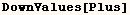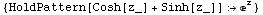Instead of associating the above identity with Plus (an often used function)
we can associate the identity with a head one level deeper than Plus.  In
this case we can associate the identity with Cosh and/or Sinh as an UpValue.
Before proceeding we should do away with the above definition.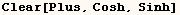Evaluating the next cell will associate the identity with Sinh as an  UpValue.  For more on UpValues see The Mathematica Book section 2.4.10.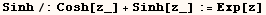By starting the line above with Sinh/:  the kernel knows to associate the
identity with Sinh.  Once the previous cell is evaluated the next cell can be
used to see that the identity is associated with Sinh.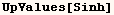At first one might guess that the above Identity would cause the next cell to
change into Exp[3.5] which then evaluates to 33.1155.  However Cosh[3.5]
evaluates to 16.5728, and Sinh[3.5] evaluates to 16.5426 before the kernel
checks for the above identity.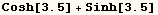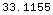Now when the next line evaluates the above identity is used, but only after
the kernel finds it can't do anything with Cosh or Sinh.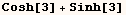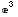Now consider evaluation of the expression below.  Here the above identity  is still stored in UpValues[Sinh] and not  in DownValues[Plus] or anywhere  else.  Since the identity isn't stored with Plus there is no impact on the  evaluation of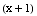,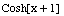,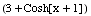or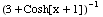.  In fact the above identity has no impact on the evaluation of  Sinh.   However, when the kernel evaluates (2 x Sinh) it checks for user defined  UpValues of Integer (the head of 2), but there are none.  Then the kernel  checks for user defined UpValues of (x), but there are none.  Then the kernel  checks the for user defined UpValues of Sinh, and there is an UpValue.  At  that point the kernel must see if the UpValue for Sinh can be used, but finds  that it can't.  Then the kernel checks for built in UpValues for Integer, x,  Sinh and either finds none or none that apply to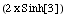.  Then the kernel checks for user defined DownValues for Times and finds  none.  Finally the kernel checks for built in DownValues for Times, and  either finds none or none that apply to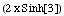.  At that point evaluation is complete.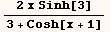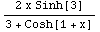Storing the identity forwith UpValues[Sinh] should have much less impact on the evaluation of  typical expressions than storing it with DownValues[Plus].  However, this  identity will have an impact on the evaluation of many expressions that  include Sinh, and this is likely the reason why Wolfram Research didn't  include it as part of the default evaluation process.

Evaluating the next  cell will remove any the above definitions.Instead of storing the above identity with UpValues[Sinh] one could store  it with UpValues[Cosh] using the next cell.  The only difference this will  make is that it will slow down evaluation some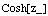expressions, but never slow down evaluation of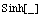expressions.  Starting the next line with Cosh/:  tells the kernel to  store the identity with UpValues[Cosh].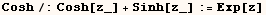Still another way to store this identity is to use the next line.  That  will store the identity with UpValues for each symbol at level 1 of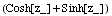.  In this case Plus is at level 0 and Sinh, Cosh are the only symbols at  level 1.  This approach will slow down evaluation of Sinh[_] and Cosh[_]  expressions with no advantage over storing the identity only with  UpValues[Sinh], or only with UpValues[Cosh].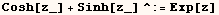All example above used UpValues with delayed assignment.  Mathematica provides ways to make UpValues with immediate assignment, but one should  be very cautious of them.  This is done in the cells below.  In each case then variable  (z) is used for the named pattern on the left side, but when (z) has a global  value this global value will be used on the right side.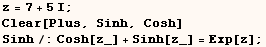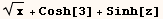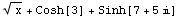Instead a definition such as the one above should be stored as an UpValue
with delayed assignment as I do in the next cell.  When delayed assignment is
used a global value assigned to (z) will not affect the results.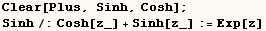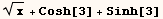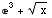We can't assign a definition with a symbol that's too deep inside an
expression.

We can use UpValues to store the identity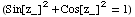.  However, this identity can't be stored with the UpValues[Sin] or  UpValues[Cos] because Sin, Cos are too deep in the expression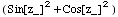.  UpValues must be stored with a symbol that appears at level 1.  In this  case Plus is at level 0, Power is at level 1, Sin, Cos, Integer are at level  2.  The identity can be stored in  UpValues[Power] as in the next cell.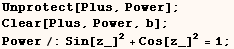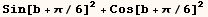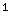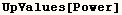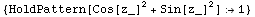We can also store the identity with DownValues[Plus] using the next cell.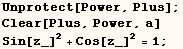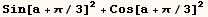Before continuing you might want to evaluate the following to remove all
definitions made above.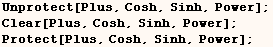Created by Mathematica  (May 16, 2004)

Back to Ted’s Tricks index page